# Taylor Series in Python

The Taylor series of a function is an infinite sum of terms that uses information about the derivative of the function to create a polynomial that approximates the function. More accurate approximations can be determined by taking higher-order derivatives and using higher degree polynomials. Many posts about the Taylor series (and some YouTube videos) help build an intuition for the infinite series by showing how higher-order terms provide ever closer approximations of the underlying function (provided the series converges). However, there aren’t many that show why we should really care.

When first confronted with the Taylor series while working on my undergraduate degrees, they seemed like just another thing. Recently, while studying for the GRE Mathematics Subject Test, I developed a deeper appreciation for the Taylor series and decided to implement them in Python. This implementation requires only the definition of the function being approximated, the rest is handled numerically. In this post, the Python implementation, along with some potential uses for the Taylor series are discussed and presented.

## Implementation

The terms of the Taylor series of a function, f(x), require successively higher-order derivatives,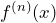, to determine the coefficients of the polynomial. Therefore, a Taylor series can only be determined for f(x) if it is infinitely differentiable. The terms of the series are given by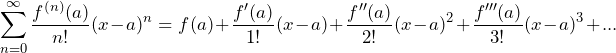Where a is the center of the Taylor series. When the center of the series is 0, i.e. a=0, the series is also called the Maclaurin series.

To be able to determine the Taylor series for a function programmatically all we really need to do is determine the coefficients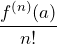for a sufficient number of terms. Note that the more terms we use in the Taylor series the more accurate the approximation will be. Fortunately, in the scipy Python package, there is a built-in function for computing the derivative of a function at a specified point. We will use this function to determine the coefficients of the polynomial.

In the logic above, we start by defining a class to store the Taylor series information. The constructor takes a pointer to a function for which we’re finding the Taylor series, the order of the Taylor series (i.e. the number of terms), and the center of the series which defaults to the Maclaurin series. Some variables are computed on the fly which are used in the scipy.misc.derivative function. Immediately, the private __find_coefficients(…) method is called to determine the coefficient values for each term in our Taylor series. This is done by numerically by determining the derivatives for each term of the Taylor series and dividing by the factorial, as is defined above.

In actuality, this is really all that needs to be done to represent the Taylor series in Python. The remainder of the capabilities of the Taylor series will just use these coefficients to perform different operations. Before getting into that, let’s verify this is working by determining the coefficients of some polynomials (the Taylor series for which should be the exact function). To verify this, two print functions are defined to view the coefficients and the resulting equation representing the Taylor series.

The first function, print_equation(…), prints the Taylor series as an equation centered at the series’ center. print_coefficients(…) will just print a list representing the coefficients and get_coefficients(…) will return this list.

The code below is used to find the coefficients of the Taylor series representing the function in the Python function f(x)

Running this logic will produce a list of size 15 that contains the coefficients of the Taylor series and will also print the polynomial equation. In this case, the function being “approximated” with 15 terms of the Taylor series is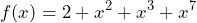Since this is just a polynomial the Taylor series should be able to represent this exactly by having a first coefficient (the constant) of 2.0, coefficients of 1.0 at indices 2, 3, and 7, and coefficients of 0.0 everywhere else. This would represent the polynomial shown above. Indeed running the script produces the following output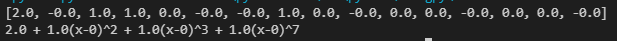The output of the Python script above shows that the coefficients are determined correctly and that the original polynomial is produced by the Taylor series.

Changing the function f(x) in the above Python script for different polynomials produced the same results, each polynomial is exactly represented by the Taylor series. This isn’t very interesting but it is a good way to verify that the logic is working as expected. More interesting functions for which the Taylor series is widely known, e.g.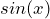,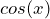,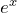, etc., also provide the correct results via this implementation. These functions will be used in the applications below.

## Applications

Because polynomials are typically easier to work with than most functions, a Taylor series approximation can help determine approximate values for different operations concerning these functions.

### Useless

#### Differentiation

The Taylor series of a function can be used to approximate the derivative of a function at a particular point. The terms in the Taylor series can be differentiated individually and take the form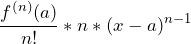which is simply the derivative of the polynomial term via the power rule multiplied by the coefficient of the Taylor series. Note that, in the code, this will weed out terms not represented by the Taylor series as the coefficient will be 0.

These calculations are done in the Python logic with the function below

In this function, the approximation of the derivative of the function is found by iterating over the coefficients, computing the derivative values as described above, and summing them up. Plugging values into this function provides an accurate approximation of the derivative of the underlying function as shown below for cos(x)

As seen here, the values 0,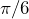,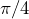,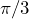,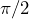, and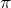are used and the functional value,and the approximated derivative are shown (note that the actual derivative is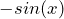). Looking at a few points we see that, in general, this gives a good approximation of the derivative ofat these points. For example, at the pointthe functional value is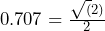is correct as is its deriviative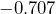.

Unfortunately, this is essentially useless since the Taylor series requires information about the derivative in order to determine its coefficients. Why would we want an approximation of f'(x) when we’re required to know the general form (thus the actual value) of f'(x) in order to determine this approximation? Also, there are many different numerical methods that can approximate derivatives without analytically finding the derivative of the function (e.g. finite-difference methods) which are more suited to this task.

### More Useful

#### Value Approxmation

One widely used purpose of the Taylor series is to approximate values of the underlying function. To approximate functional values, the value is plugged into the Taylor series terms which are added together. This is done in the Python logic below.

#### Definite Integration

The Taylor series can be used to approximate the integral of the underlying function since the terms of the Taylor series can be integrated individually, as was done with differentiation. The terms in the approximation of the integral will be of the form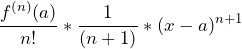Here again, this is just the power rule for integration multiplied by the Taylor series coefficient.

However, numerically, we can only determine the definite integral of the function since, otherwise, the lack of a value for the constant of integration can lead to incorrect results. Consider the Taylor series for f(x) = sin(x) centered at 0: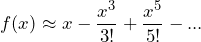integrating this term by term provides the following polynomial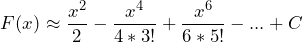Now, assume this is an appropriate approximation of the integral of sin(x) (for which we know the actual integral is -cos(x)) and try to evaluate this function at 0. The value from doing so is 0. In this case, this can be remedied by setting the constant of integration C=-1 but we would have to determine this constant for every value in the function’s domain just to “fix” the integrals which makes indefinite integration kind of useless.

Definite integrals on the other hand can easily be computed by integrating the Taylor series term by term and plugging in the limits of integration, this is shown in the Python code below.

Running this for a few values provides the desired results for,,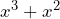,, and, one that is a little more difficult to integrate,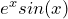. Due to the length of this post, these results will be omitted. For those curious, the full code can be used below and the def f(x) function can be changed to see these results for yourself.

#### Limits

Rather than showing how to approximate limits numerically and implementing that in Python, I will just go over an example of a limit that might be difficult to determine analytically but can easily be found by substituting in a Taylor series.

Consider the limit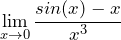This limit can easily be determined via repeated application of L’Hosptials rule as it has the form 0/0 but let’s assume for a minute that we don’t know that (or that we don’t know anything about L’Hospitals rule), then how would we determine this limit? It turns out that the Taylor series can help us with this by replacing sin(x) in the limit with an approximation. For this example, a 3-term Taylor series will be used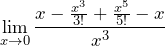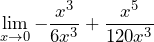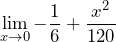Since the term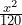goes to 0 in the limit the result is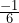, as expected if evaluated via L’Hospitals.

## Conclusion

Introduced above was the idea of the Taylor series which is a mathematical tool used to approximate any continuously differentiable function with a polynomial using only information about the function’s derivative. A Python implementation was provided and some applications of the Taylor series were discussed. The full code with some example usage is provided below which I encourage anyone interested in this tool to copy and play with to gain a further understanding of the Taylor series.